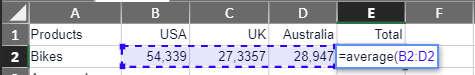# Working with Formulas

Tabulations frequently contain data from various queries, necessitating the merging of information from these diverse sources. Formulas play a vital role in tabulations as they enable you to perform calculations, retrieve specific data, manipulate cell contents, evaluate conditions, and accomplish much more.

#### Create a formula using cell references:

1. Select the cell where you want to enter the formula.
2. Type = (equal sign) to start the formula.
3. Enter the formula by typing the first cell reference. For example, to add up the values in cells A1 and A2, type A1.
4. Enter the operator. +
5. Select the second cell, or type A2
6. Press Enter to apply the formula to the selected cell.
7. The result of the formula will be displayed in the cell.
8. The formula is displayed in the formula bar

#### Create a formula using a built-in function:

1. Select the cell where you want to enter the formula.
2. Type = (equal sign) to start the formula.
3. Enter the function. For example, to calculate the average of the values in cells B2 to D2, type Average
4. Type an opening round bracket: (
5. Select the cells B2 to D2
6.7. Type a closing round bracket )
8. Press Enter to apply the formula to the selected cell.
9. The result of the formula will be displayed in the cell.
10. The formula is displayed in the formula bar